##### Ti-84 Plus Graphing Calculator For Dummies, 2nd EditionBefore you can graph a function on your TI-84 Plus calculator, you must enter it into the calculator. The calculator can handle up to ten functions at once, Y1 through Y9 and Y0. To enter functions in the calculator, perform the following steps:
1. Press [MODE] and put the calculator in Function mode.

To highlight an item in the Mode menu, use the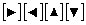keys to place the cursor on the item and then press [ENTER]. Highlight FUNCTION in the fourth line to put the calculator in Function mode. See the first screen.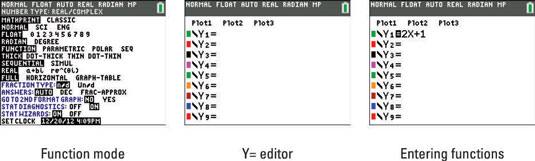2. Press ! to access the Y= editor.

See the second screen.

If necessary, press [CLEAR] to erase a previous function entry. Then enter your function.

Your math textbook may use a function notation like this: f(x)=2x+1. To graph a function in your calculator, you must realize f(x) is interchangeable with y, only the notation differs. See the third screen.

When you’re defining functions, the only symbol the calculator allows for the independent variable is the letter X. Press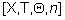to enter this letter in your function.

As a timesaver, when entering functions in the Y= editor, you can reference another function. Use the shortcut Y-VAR menu to paste a function name in the function you’re entering in the Y= editor. Just press [ALPHA][TRACE] and choose the name of the function you want to insert in your equation. See the first screen.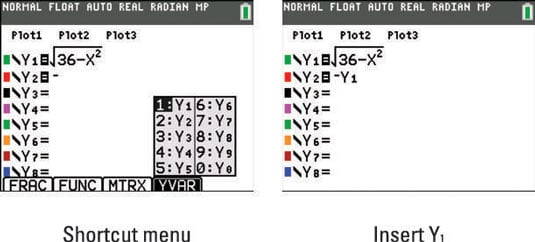How does calling up the name of another function save you time? Well, say you’re trying to graph a circle in your calculator with the equation x2 + y2 = 36. Of course, you need to solve the equation for y to graph the circle equation in your calculator. Solving for y gives you: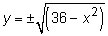Notice, it takes two functions to graph a circle! No problem. In function Y1 enter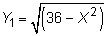Then, to save time, use the shortcut YVAR menu to enter Y2 = Y1. See the second screen.